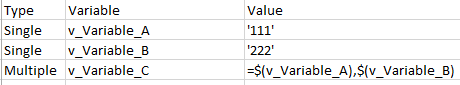# QlikView App Dev

Discussion Board for collaboration related to QlikView App Development.

Announcements
Our next Qlik Insider session will cover new key capabilities. Join us August 11th REGISTER TODAY
cancel
Showing results for
Did you mean:Not applicable

## Having one variable set to multiple variable values

Hello,

Somewhat new to QV and couldn't figure this one out.I am loading variable values from a spreadsheet by looping through an excel file.

Command,
Variable,

Value

From Excel File

When I use run this logic with the below excel file, I get v_Variable_C = '111' just as I am hoping forHowever, I am confused on how to do this when v_Variable_C equals multiple variables. This setup doesn't seem to work. Is there some modification I can make in my load to where v_Variable_C = '111','222'?3 RepliesMVP

LET vVariable_C = '\$(v_Variable_A)' & ',' & '\$(v_Variable_B)' ;

talk is cheap, supply exceeds demandNot applicable
Author

Thanks. This solution would essentially require us to hardcode the value for Variable C. Is there anyway where we can detect if there is multiple variables (perhaps trigger off that Type column) to do this in a more automated sense?Partner

Hi,

try this:

Data:

Type;Variable;Value

Single;vVarA;'111'

Single;vVarB;'222'

Multiple;vVarC;\$(vVarA),\$(vVarB)

(Note the missing '=', for vVarC)

```Var:
Variable,
Value
FROM / Resident / ... ;

SVar:
NoConcatenate
Where Type = 'Single';

for i=0 to NoOfRows('SVar')

let _Name = Peek('Variable', i, 'SVar');
let \$(_Name) = Peek('Value', i, 'SVar');

NEXT;

drop table SVar;

MVar:
NoConcatenate
Variable,
Concat(Value, '&') as Value
Group By Variable;
Variable,
SubField(Value, ',') as Value
Resident Var
Where Type = 'Multiple';

for i=0 to NoOfRows('MVar')

let _Name = Peek('Variable', i, 'MVar');
let _Value = Peek('Value', i, 'MVar');
let \$(_Name) = \$(_Value);

NEXT;

let _Name = null();
let _Value = Null();

drop table MVar;
```

this creates the variable vVarC with the Value 111222. If you change vVarA or vVarB in the QV-App vVarC wil not be changed.

If you want that vVarC chanes if you alter vVarA or vVar use this code to create the 'Multiple' Variables:

```for i=0 to NoOfRows('MVar')

let _Name = Peek('Variable', i, 'MVar');
let \$(_Name) = Peek('Value', i, 'MVar');

NEXT;
```

If you use this code, you have to use \$(vVarC) to get the Value.

Regards

Sebastian LettnerCommunity Browser